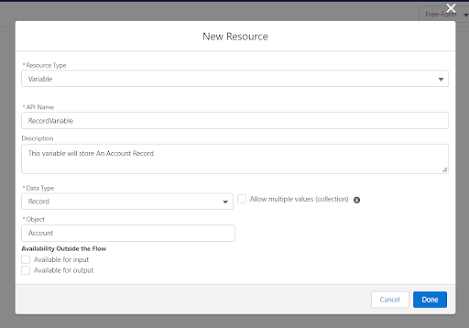# EP - 23 | DIFFERENCE BETWEEN RECORD VARIABLE, TEXT VARIABLE AND RECORD COLLECTION VARIABLE | LIGHTNING FLOW SERIES⚡️

## Difference Between Record Variable, Text Variable and Collection Variable

In this blog you will learn difference between record variable, text variable and collection variable.
So to some of you this will be a very basic and simple thing but for many of you this is a main confusing topic in flow while creating variable.

So before going any further in learn flow I thought let's relax, take a deep breathe and go back to basics.

Let's first talk about what is the difference between Record Variable and Text Variable.

What is Text Variable?
So, let's say if you want to store a text of string what we do in coding is we take a string variable and store our string into that.
Same as in flow if we want to store a string value we will create a text(string) variable.
How to define if it is a text variable. To create a Text Variable we will have to set the "Data Type" option to "Text".

Here is the example :

There are other options also. If you want to set the default value of the variable. If you want to set the variable for input or output.

Now Let's understand about record variable.

What is Record Variable?
So, If you want to store an Object's record. For example if you want to take a "Account" record in apex what we will write is :

Account accounts = [Select Id, Name, Anything else from Account limit 1];

``Same as if we want to store an Account record in flow we will use Record Variable.``

``Here is the example :``

``Here the "``Data Type``" will be "``Record``" because we want to save a record in that.``
`Once you will select "Record" it will ask you to select the object.`

`So that is the difference between Text and Record Variable.`

`Now let's learn about Collection Variable.`

``What is a Collection Variable?``
``So, let's say you want to store a single record then you take Record Variable. ``
``But what if you want to store list of Records. Will you take multiple variables for that?``
``If you are saying yes, then think again.``

`So, if we want to store list of records then we will just create a Record Variable same as shown above. `
`But we will check the checkbox which says "Allow multiple values(collection)".`
`When you select this that means you can store multiple values in that variable.`

`Have a look :`

I hope this blog have helped you in understanding there three very important things.

To learn it in detail checkout this video link :